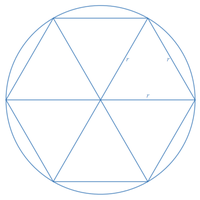# Help me with this!

#### MarkFL

##### Super Moderator
Staff member
I would observe that, if we call $$r$$ the radius of the circle in which the regular hexagon can be inscribed, then:

$$\displaystyle \overline{HI}=\frac{1}{2}r$$

And:

$$\displaystyle 2\overline{FI}+\overline{HI}=2r$$

See what you can do with that...•topsquark

#### MarkFL

##### Super Moderator
Staff member
To add a bit of clarity to my previous post, consider the following diagram of a regular hexagon inscribed within a circle of radius $$r$$, and decomposed into 6 equilateral triangles having side lengths $$r$$:Now, in your diagram, we can see that $$\triangle GHI$$ and $$\triangle GDE$$ are similar, with the latter having twice the altitude of the former. And so the base of the latter, which is $$r$$ will be twice the base of the former.

The rest of what I previously posted now follows.